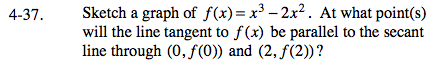### Home > CALC > Chapter 4 > Lesson 4.1.3 > Problem4-37

4-37.Calculate the slope of the secant between (0, f(0)) and (2, f(2)).

We want to know where the slope of the tangent is the same as the slope of the secant. Recall that the slope of the tangent is also
known as f '(x), find where f '(x) = 0.

The slope of the tangent = the slope of the secant at coordintate points ( ________, _________ ) and ( ________, _________ ). You must analytically compute the exact coordintaes, but note that the slope of tangents lines is 0 at the local maximum and local minimum.

Use the eTool below to examine the graph.
Click the link at right for the full version of the eTool: Calc 4-37 HW eTool